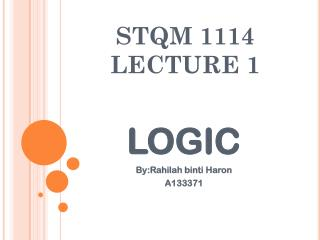DownloadDownload PresentationSTQM 1114 LECTURE 1

# STQM 1114 LECTURE 1

Download Presentation## STQM 1114 LECTURE 1

- - - - - - - - - - - - - - - - - - - - - - - - - - - E N D - - - - - - - - - - - - - - - - - - - - - - - - - - -
##### Presentation Transcript

1. STQM 1114LECTURE 1 LOGIC By:RahilahbintiHaron A133371

2. Objectives • Be able to identify a statement and compound statements • Be able to use sentential connectives/logical operators in words and symbols • Be able to summarize statements in a truth table • Be able to use quantifiers in a statement • Be able to perform simple proof using logical statements

3. Part 1:logical connectives • The study of critical thinking or reasoning (taakulan), is called logic • All reasoning is based on the ways we put sentences together • The languages of mathematics consists primarily of declarative sentences. • If a statement can be classified as true or false, it is called as statement. • The truth or falsity of a statement is known as its truth value

4. What is statement??? • A statement is denoted with a small letters p,q,r • The truth value is usually given in a Truth Table • Symbol F (false) and T (true) • Example: • a)Three plus two is five - A statement since it is true • b)”Did you get that one right?” -Not a statement since it is a question • c)All triangles -Not a statement.It is a phrase

5. Compound statement • A new statement that combines one or more statements using the logical operator /sentential connectives • Example: • Today is warm and yesterday was cold -It is made up of two parts:”Today is warm” and “yesterday was cold” • These two parts can also be combined in others ways. • Examples: • Today is warm or yesterday was cold • If today is warm then yesterday was cold • Today is warm if and only if yesterday was cold

6. Logical Operator: • ˆ:for “and”/conjuction • ˇ: for “or”/disjunction • ~:for “not”/negation • →:for “if….then”/implication or conditional statement • ↔:for “if and only if”/equivalence

7. Negation:not Let p stand for a given statement Then ~p (read not p) represents the logical opposite (negation)of p When p is true, then ~p is false; when p is false, then ~p is true.This can be summarized in a truth table:

8. Conjuction : and Example: 1.Blue is a color and 7+3=10 (T and T=True) Since both facts are true ,the entire sentence is true 2.”One hour=exactly 55 minutes and one minute=exactly 60 seconds”(F and T=False) Since the first fact is false,the entire sentence is false

9. Disjunction:or Examples: 1.Blue is a color or 7+3=10 (T or T=True) Since both facts are true ,the entire sentence is true 2.”One hour =exactly 55 minutes or one minute=exactly 60 seconds (F or T=True) Since the second fact is true,then the entire sentence is true

10. Conditional : if …..then A statement of the form If p……………then q is called an implication or a conditional statement.The if-statement p in the implication is called the antecedent (hypothesis) and then-statement q is called the consequent(conclusion)

11. Some of movies that have relations with logic.. Watch it..^^ • http://www.math.harvard.edu/~knill/mathmovies/swf/shrek3_lies.html • http://www.math.harvard.edu/~knill/mathmovies/swf/maandpakettleaddition.html

12. With that… THANK YOU….